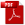Download as PDFProof that almost all numbers $n$ are composed of about $\log \log n$ prime factors
Proceedings of the London Mathematical Society, 2, XVI,1917, Records for 14 Dec. 1916

A number $n$ is described in popular language as a round number if it is composed of a considerable number of comparatively small factors: thus $1200=2^4\cdot 3\cdot 5^2$ would generally be said to be a round number. It is a matter of common experience that round numbers are exceedingly rare. The fact may be verified by anybody who will make a practice of factorising numbers, such as the numbers of taxi-cab or railway carriages, which are presented to his attention in moments of leisure. The object of this paper1 is to provide the mathematical explanation of this phenomenon.

Let $\pi _{\nu}(x)$ denote the number of numbers which do not exceed $x$ and are formed of exactly $\nu$ prime factors. There is an ambiguity in this definition, for we may count multiple factors multiply or not. But the results are substantially the same on either interpretation.

It has been proved by Landau2 that

\begin{equation} \pi_{\nu }(x)\sim \frac{x}{\mbox{log }x}\frac{(\mbox{log log }x)^{\nu -1}} {(\nu -1)!}, \end{equation}
as $x\to \infty$, for every fixed value of $\nu$. It is moreover obvious that
\begin{equation} [x]=\pi _1(x)+\pi _2(x)+\pi _3(x)+\cdots , \end{equation}
and
\begin{equation} x=\frac{x}{\mbox{log }x}\left \{ 1+\mbox{log log }x +\frac{(\mbox{log log }x)^2}{2!}+\cdots \right \}. \end{equation}

Landau's result shews that there is certain correspondence between the terms of the series (2) and (3). The correspondence is far from exact. The first series is finite, for it is obvious that $\pi _{\nu }(x) =0$ if $\nu >(\mbox{log }x/\mbox{log }2$; and the second is infinite. But it is reasonable to anticipate a correspondence accurate enough to throw considerable light on the distribution of the numbers less than $x$ in respect of number of their prime factors.

The greatest term of the series (3) occurs when $\nu$ is about $\mbox{log log }x$. And if we consider the block of terms for which

\begin{equation} \mbox{log log }x -\phi (x) \sqrt{(\mbox{log log }x )} \lt \nu \lt \mbox{log log }x +\phi (x) \sqrt{(\mbox{log log }x )}, \end{equation}
where $\phi (x)$ is any function of $x$ which tends to infinity with $x$, we find without difficulty that it is these terms which contribute almost all the sum of the series: the ratio of their sum to that of the remaining terms tends to infinity with $x$.

In this paper we shew that the same conclusion holds for the series (2). Let us consider all numbers $n$ which do not exceed $x$, and denote by $x_P$ the number of them which possess a property $P(n,x)$: this property may be a function of both $n$ and $x$, or of one variable only. If then $x_P/x\to 1$ when $x\to \infty$, we say that almost all numbers less than x possess the property P. And if $P$ is a function of $n$ only, we say simply that almost all numbers possess the property $P$. This being so, we prove the following theorems.

1. Almost all numbers $n$ less than $x$ are formed of more than $$\log \log x-\phi (x) \sqrt{(\log \log x )}$$ and less than $$\log \log x+\phi (x) \sqrt{(\log \log x )}$$ prime factors.
2. Almost all numbers $n$ are formed of more than $$\log \log n-\phi (n) \sqrt{(\log \log n )}$$ and less than $$\log \log n+\phi (n) \sqrt{(\log \log n )}$$ prime factors.

In these theorems $\phi$ is any function of $x$ (or $n$) which tends to infinity with its argument: and either theorem is true in whichever manner the factors of $n$ are counted. The only serious difficulty in the proof lies in replacing Landau's asymptotic relations (1) by inequalities valid for all values of $\nu$ and $x$.

Since $\log \log n$ tends to infinity with extreme slowness, the theorems are fully sufficient to explain the observations which suggested them.

Endnotes

1The paper has been published in the Quarterly Journal of Mathematics, Vol. XLVIII, pp. 76 – 92[No. 35 of this volume].

2See Handbuch der Lehre von Verteilung der Primzahalen, pp. 203 – 213.